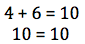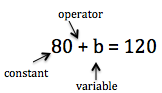# Equation Lesson for Kids: Definition & Examples

An error occurred trying to load this video.

Try refreshing the page, or contact customer support.

Coming up next: Exponent Definition: Lesson for Kids

### You're on a roll. Keep up the good work!

Replay
Your next lesson will play in 10 seconds
• 0:04 Party Planning
• 0:25 What Is an Equation?
• 1:29 Solving Equations
• 2:25 Practice Solving Equations
• 3:18 Lesson Summary
Save Save

Want to watch this again later?

Timeline
Autoplay
Autoplay
Speed Speed Audio mode

#### Recommended Lessons and Courses for You

Lesson Transcript
Instructor: Kelley Lipke

Kelley has been teaching middle school for six years and has a master's degree in educational administration.

In this lesson you will learn about mathematical equations. You will find out what equations are composed of and how to solve them. There will also be a practice problem for you to try!

## Party Planning

Max is planning a party for his friend and has been given $120 to spend on decorations. He has already spent$80 on balloons and wants to buy a box of streamers also. If streamers cost \$32 a box, will Max have enough money to buy them?

To determine if he has enough money for the streamers, Max needs to write and solve an equation.

## What Is an Equation?

An equation is a mathematical sentence that has two equal sides separated by an equal sign.

4 + 6 = 10 is an example of an equation. We can see on the left side of the equal sign, 4 + 6, and on the right hand side of the equal sign, 10.When solving math problems, like figuring out how much money Max has left to spend, a piece of the equation is going to be missing. The equation for Max's problem will look like this:As you can see, equations can also have constants, coefficients, variables, and operators.

Constants are numbers that do not change. 80 is the constant in this example.

A coefficient is a number attached to a variable. Coefficients are used in multiplication equations. For example, 12 is the coefficient in the equation 12n = 24.

A variable is a letter that represents an unknown number. In this problem, b is the variable and is the part we need to solve for.

An operator tells you which operation to use and includes addition, subtraction, multiplication, and division.

## Solving Equations

To solve this equation, Max needs to get the variable on one side by itself. He does this by using inverse operations. Inverse operations are like opposites. Whatever operation we see in an equation, we use the inverse of that operation to solve. In this case, the operator is an addition sign. So we will use the inverse operation, subtraction, to isolate the variable.

Also, because equations have an equal sign, they must always remain equal and balanced. In other words, whatever we do to one side of the equation, we need to do the same to the other side. Let's try it!

To unlock this lesson you must be a Study.com Member.

### Register to view this lesson

Are you a student or a teacher?

#### See for yourself why 30 million people use Study.com

##### Become a Study.com member and start learning now.
Back
What teachers are saying about Study.com

### Earning College Credit

Did you know… We have over 200 college courses that prepare you to earn credit by exam that is accepted by over 1,500 colleges and universities. You can test out of the first two years of college and save thousands off your degree. Anyone can earn credit-by-exam regardless of age or education level.